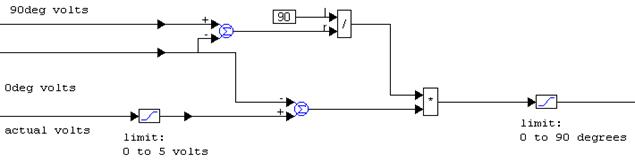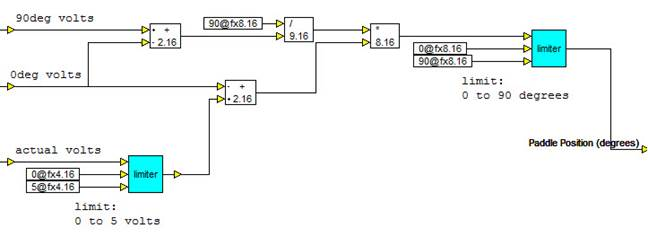##### Constructing the Fixed-Point Volts to Degrees Converter

The floating-point implementation of the Volts to Degrees Converter was the arithmetic implementation of the equation:

actual angle = (actual voltage0deg voltage) * (90deg voltage0deg voltage) / 90

The actual implementation is:This relationship can be easily implemented using the fixed-point blocks sum, div, mul, limit, and const.This set of blocks is encapsulated in the Volts to Degrees (FIXED POINT) compound block.